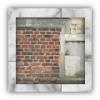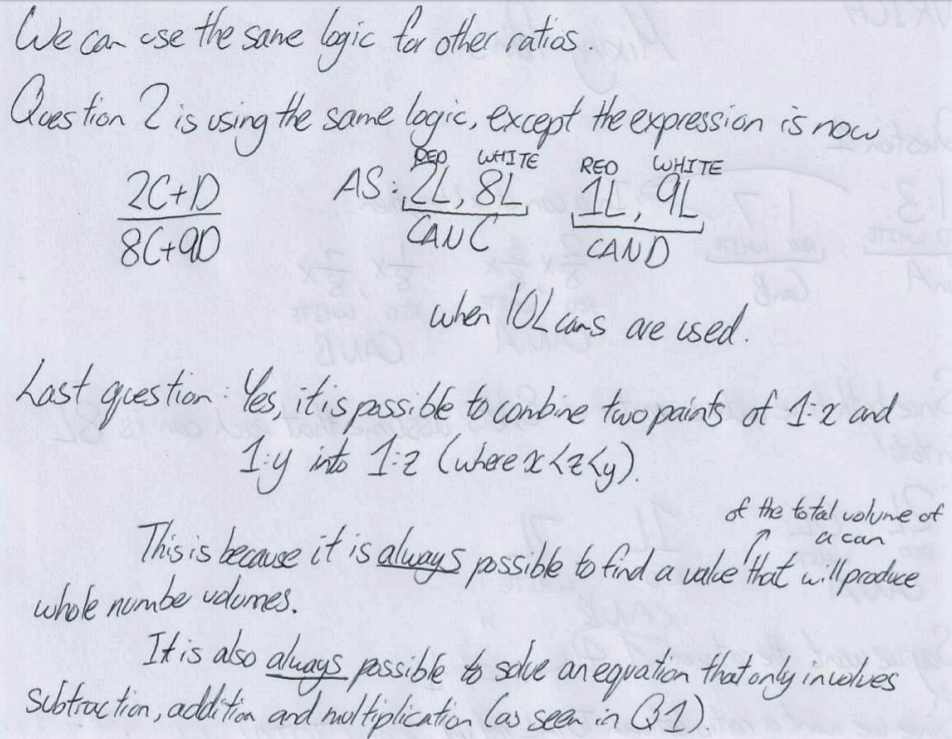#### You may also like### Rule of Three

If it takes four men one day to build a wall, how long does it take 60,000 men to build a similar wall?### Square Pegs

Which is a better fit, a square peg in a round hole or a round peg in a square hole?### How Big?

If the sides of the triangle in the diagram are 3, 4 and 5, what is the area of the shaded square?

# Mixing Paints

##### Age 11 to 14Challenge Level

TK and Stewart from Bangkok Patana School in Thailand warned of some calculations which would give incorrect results:

Some may try to just add together 1  +  1 red  :   3  +  7 white

This would be incorrect as the sum of 1 and 3 is 4; the sum of 1 and 7 is 8. 4$\neq$8 (so the cans of paint would not have been the same size).

This method is incorrect. You would not add $\frac{1}{3}$ and $\frac{1}{7}$ by adding the numerators and denominators together; it makes no sense to add together the numbers left of the colon and those right of it to create a new ratio.

Here is another way to go about solving this problem.

We know that one can contains 1 litre of paint.

In the instance of Can A, the ratio is 1:3
This means that there is 250ml of red paint in Can A and 750ml of white paint.

In Can B the ratio is 1:7
From this, we know that there is 125ml of red paint in Can B and 875ml of white paint.

If we were to mix a Can of A and a Can of B, we would have 375ml of red paint and 1625ml of white paint.

Deana, TK and Stewart from Bangkok Patana School, Moon from British International School Phuket in Thailand, Gabriel and Wooyong from BSKL in Malaysia and Abraham from Harrow International School Hong Kong worked out the quantites of Paint A and Paint B needed to get a ratio of 1 : 4. This is Moon's work:

Take 80 litres to be in the cans therefore:

Paint Can A has

Red: White
$20 : 60$

Paint Can B

Red: White
$10 : 70$

And a combination of cans is given by:

$x$(Can A) + $y$(Can B)

So the total red amount is given by $20x + 10y$
And the total white amount is given by $60x + 70y$

i.e the ratio is

Red              White
$20x + 10y : 60x + 70y$

So if we want a ratio of $1:4$ this means we have the equation:

$4(20x + 10y) = 60x + 70y$
$\Rightarrow 2x = 3y$

the LCM of $2$ and $3$ is $6,$ so we solve this by $x = 3$ and $y = 2.$

i.e we need $3$ Can As and $2$ Can Bs.

Gabriel used a slightly different method. This is Gabriel's work to find quantities of Paint A and Paint B to get a red:white ratio of 1:5.
Paint A has a red to white ratio of 1:3 and paint B has a red to white ratio of 1:7. Paint A is $\frac14$ red and $\frac34$ white and paint B is $\frac18$ red and $\frac78$ white. By mixing $x$ cans of paint A and $y$ cans of paint B, we get paint with a red to white ratio of $\left(\frac x4+\frac y8\right)$ to $\left(\frac{3x}4+\frac{7y}8\right)$ or $(2x+y)$ to $(6x+7y).$

We need to find integers $x$ and $y$ such that $\dfrac{\frac x4+\frac y8}{\frac{3x}4+\frac{7y}8}=\frac15.$

To do that, plot $\dfrac{\frac x4+\frac y8}{\frac{3x}4+\frac{7y}8}=\frac15$ and find the first point $(x,y)$ at which $x$ and $y$ are integers.
For 1:5, the point is $(1,2) \Rightarrow$ solution is 1 can of paint A and 2 cans of paint B.
Wooyong moved straight on to the ratio $1:k$ for red paint : white paint.
For any value of k, we use the added ratio, $\frac14x + \frac18y : \frac34x + \frac78y$, to solve it (by forming the equation $k\left(\frac14x + \frac18y\right) = \frac34x + \frac78y$).